Articles

# fitplc - an R package to fit hydraulic vulnerability curves

## Abstract

We describe a toolkit to fit hydraulic vulnerability curves, such as the percent loss of xylem hydraulic conductivity ('PLC curves') as a function of the water potential. The toolkit is implemented as an R package, and is thus free to use and open source. The package fits the Weibull or sigmoidal function to measurements of PLC, conductance or conductivity, at corresponding leaf or stem water potentials. From the fitted curve, estimates of Px (the water potential at which x% conductivity is lost, e.g. the P50), and slope parameter (Sx) are provided together with confidence intervals (CI) around the fitted line. The CIs are estimated with the bootstrap. We also demonstrate the advantages of using mixed-effects models in situations where multiple individuals are measured on a species, as compared to the more traditional approach of fitting curves separately and averaging the parameters. We demonstrate the use of the new package with example data on seven species measured with two different techniques.

## Introduction

Water is transported through the xylem under tension and is thus prone to cavitation, the rapid phase change from liquid to water vapour (Dixon and Joly, 1895; Tyree and Sperry, 1989). This results in the formation of gas bubbles (emboli) that block xylem conduits and reduce the hydraulic conductivity of the xylem. The probability of embolism formation is greatly increased by environmental stresses such as drought. During drought, the tension necessary to draw water from the soil increases as soil water content declines. At some critical threshold, embolism begins to spread through the xylem network via air seeding of pit membranes (Tyree and Zimmermann, 2013; Choat et al., 2015). During extreme droughts, embolism blockages can cause complete hydraulic failure in the xylem pathway and subsequent death of the plant (Kursar et al., 2009; Urli et al., 2013; Li et al., 2016a). Indeed, hydraulic failure is now considered to be a principal cause of tree mortality during drought (Brodribb and Cochard, 2009; Anderegg et al., 2016). This is particularly relevant since the hydraulic system of plants is finely tuned to their growing environment with the majority of woody plant species across major forest biomes maintaining narrow safety margins to hydraulic failure (Choat et al., 2012). Given the importance of vulnerability to embolism for plant survival during periods of environmental stress, it is essential that appropriate statistical methodology is developed for comparison of between and within species.

Plants vary widely in their vulnerability to embolism with vulnerability strongly related to the minimum seasonal water potential experienced in the field (Choat et al., 2012). This variation in vulnerability results from differences in xylem anatomy, principally the physical characteristics and distribution of inter-conduit pit membranes (Tyree et al., 1994; Choat et al., 2008; Pittermann et al., 2010; Li et al., 2016b). Vulnerability to embolism is described by the relationship between loss of hydraulic conductivity in the xylem and xylem water potential, which produces a vulnerability curve (Fig. 1). Because of the importance of the trait to survival under conditions of drought stress, it is common to investigate differences in vulnerability to embolism among species, populations, plant components or experimental treatments. Vulnerability is compared using the xylem water potential at which some fixed proportion of hydraulic conductivity is lost, most commonly 50%, (known as the P50) although other points are also used, e.g. Pe, P25, P88, P95 (Tyree and Ewers, 1991; Tsuda and Tyree, 1997; Brodribb and Hill, 1999; Sparks and Black, 1999; Choat et al., 2003; Schuldt et al., 2015). These parameters are determined by fitting a response curve to the data for each species, population or organ.

A range of methods are now employed to generate vulnerability curves and can generally be divided into two classes; those that expose a sample (branch, root, leaf) to repeated measurements and those in which each sample is only measured once. For instance, the bench dehydration method uses a collection of individual samples to construct a single composite curve (Sperry et al., 1988; Tyree et al., 1993). In techniques based on air injection, centrifugation, and imaging repeated measurements of percentage loss hydraulic conductivity or percent embolism can be made at different xylem pressures (Cochard et al., 1992; Cochard et al., 2005; Pockman et al., 1995; Choat et al., 2015). Vulnerability curves have been fitted to these data using a variety of functions including sigmoidal, Weilbull and polynomial (Pammenter and Van der Willigen, 1998; Ogle et al., 2009).

Clearly, when making comparisons, statistical testing of the differences in P50 is not possible unless the confidence interval (CI) is reported. The widely used method by Pammenter and Van der Willigen (1998) does not discuss the CI of P50 (or of the slope parameter), but instead recommends that statistical comparisons are made based on replicate curves, not based on the uncertainty of the parameter estimates from individual curves. In many cases, however, it is not possible to collect many replicate curves because of the time consuming nature of measurements or limitations in plant material available for destructive harvests. It is therefore also useful to report the CI of the fitted parameters for each individual curve, which allows statistical comparison across different groups based on composite curves.

In many cases the uncertainty of vulnerability curve parameter estimates has not been reported in the plant hydraulics literature, and yet conclusions have been drawn on apparent differences between groups. Indeed, the widely used method by Pammenter and Van der Willigen (1998) does not discuss confidence intervals or standard errors of P50 (or of the slope parameter - see Methods). A hierarchical Bayesian method proposed by Ogle et al. (2009) estimates full uncertainties of the parameters but is difficult to implement and has not seen widespread uptake by the field. Apart from uncertainty of the parameters that describe the curve, it would also be very useful to be able to compute confidence intervals along the entire fitted curve, in order to judge statistical significance of differences between curves at any value of the water potential.

Here we present an open source implementation of a fitting routine that can be used in estimating P50 (or any other point on the curve) and its statistical uncertainty. There is a clear need for such a utility to allow for fully reproducible results in plant hydraulics research. The fitplc package, a toolkit written in the widely used R language, is the first open source toolkit that fits PLC curves, and is easy to use and install. We have previously used and briefly described an old version of this package (Nolf et al., 2015). Here we describe the current functionality in detail, and demonstrate its use with several examples.

## Materials and Methods

Hydraulic vulnerability parameters

The fitplc package fits non-linear curves to measurements of 'percent loss of conductivity' (PLC) or raw conductance/conductivity (K) at corresponding leaf or stem water pressure (P). From these curves, two parameters are estimated: the Px (the value of P where x% of the conductivity is lost, for example P50) and a slope parameter, i.e. the derivative (Sx, % MPa-1). The package provides the choice of two models to fit the data: the Weibull (as reparameterized by Ogle et al. (2009)), and a sigmoidal model (Pammenter and Van der Willigen, 1998).

The user either provides the data as PLC, or as K. Either variable is first converted to the conductance relative to the maximum value (K/Kmax) as,

$PLC=\frac{{K}_{max}-K}{{K}_{max}}\cdot 100$
(1)

and

$K={K}_{max}*\left(1-\frac{PLC}{100}\right)$

Using the above relationships between the variables, it is straightforward to express any measurement as the relative conductance (K/Kmax).

The Weibull model

The Weibull model is given by Eq. 2, where K/Kmax is expressed as a function of P, and the two parameters to be estimated (Px and Sx).

$K/{K}_{max}=\left(1-x/100{\right)}^{p}$
(2)

where

$p=\left(P/{P}_{x}{\right)}^{{P}_{x}{S}_{x}/V}$

where

$V=\left(x-100\right)log\left(1-x/100\right)$

where Px is the xylem pressure (P) where x% of the conductivity is lost, Sx is the derivative (% MPa-1) at x (e.g. S50 is the slope of the curve at P50). Higher values of Sx thus indicate a steeper response to P. This reparameterization from Ogle et al. (2009) allows a straightforward implementation as a non-linear regression model, thus enabling computation of standard errors with textbook methods. We use ordinary non-linear least squares (with the 'nls' function in R) to fit Eq. 2 to observations of either PLC or K (converting either to K/Kmax based on Eq. 1) measured at a range of water potentials (P). Confidence intervals (CIs) of the fitted parameters are estimated using standard profiling methods (Ritz and Streibig, 2008), and with the bootstrap (see below).

Because we use non-linear regression for the Weibull model, suitable starting values have to be estimated in order for the solution to converge. We estimate starting values for Px and Sx from the fit of the sigmoidal model, which can be fit using linear regression and thus always yields a solution.

The sigmoidal model

We have also implemented a sigmoidal-exponential model proposed by Pammenter and Van der Willigen (1998), as follows. The equation is,

$PLC=\frac{100}{1+exp\left(a\left(P-b\right)\right)}$    (3)

Eq. 3 can be linearized to allow use of linear regression (Eq. 4),

$log\left(100/PLC-1\right)=aP-ab$        (4)

Eq. 4 can be directly used in a linear regression, yielding ab (as the intercept), and a (as the slope), from which Px can be estimated as

${P}_{x}=log\left(1/\left(1-x/100\right)-1\right)/a+b$    (5)

Finally, the slope parameter Sx is found as the derivative of Eq. 3 evaluated at Px (equation not shown for brevity). Because the parameters Px and Sx are non-linear parameter combinations, we report only the bootstrap confidence intervals for the sigmoidal model (these will be more appropriate anyway, given there is often non-constant error variance in hydraulic vulnerability curves, see discussion by Ogle et al. (2009)).

Bootstrap confidence intervals

We implemented the non-parametric bootstrap (Efron and Gong, 1983) to estimate confidence intervals for the estimated parameters, both for the Weibull and sigmoidal models. A common problem with bootstrapping non-linear regression models is that they do not always converge, which limits application to smaller sample sizes (Fox, 2002). We avoid this problem by estimating Px and Sx using the sigmoidal model first, and use these as accurate starting values, thus permitting bootstrap confidence intervals even with small sample sizes.

With the bootstrap, the original data are resampled with replacement N times (typically, N = 1000), and each time the regression is applied to the resampled dataset to provide estimates of the fitted parameters as well as the fitted curve at a range of values of P. The bootstrap was implemented as a simple non-parametric 'case resampling' method very similar to that described in the 'bootCase' function in the ‘car’ package (Fox and Weisberg, 2010).

Random effects

When fitting an ensemble of curves, for example when measurements were made on many branches for a single individual or species, we use a (non-)linear mixed effects (LME) model approach (Pinheiro and Bates, 2006). In this situation, it would not be appropriate to combine all individual data points and fit a single curve, as measurements are correlated within branches (and thus, the SE will typically be underestimated). Instead, the common approach is to fit curves to each branch separately, and average the coefficients. However, in some cases the data do not lend themselves well to allow curve fitting on each branch separately (in particular, when some individual branches show a very poor fit). In addition, LME will be in general more robust to individuals with poor data (Pinheiro and Bates, 2006). A similar approach was taken by Peek et al. (2002) when fitting non-linear curves of the response of photosynthesis to light.

Using LME, we obtain a single population average curve (the fixed effect) as well as the branch-to-branch variance in Px and Sx. Also estimated are the individual random effects (the so-called BLUPs, see Robinson (1991)), which can be used to visualize the differences between individual branches within the individual. The BLUPs are more robust than fitting curves to each branch separately particularly when some individuals show a poor fit. For the Weibull model, we use a non-linear mixed-effects model, and for the sigmoidal model a linear mixed-effects model. Both are fit using the ‘nlme’ R package (Pinheiro and Bates, 2006).

Weights

Following the discussion by Nolf et al. (2015), we also allow for the specification of a weighting function. Nolf et al. argued that the data around the P50 should be weighted more heavily, when we are primarily interested in the fit of the curve in that region. We have made no a priori choice on the form of the weighting function, but allow for its specification when fitting the Weibull or sigmoidal models, and suggest more research is needed on the correct choice of the weighting function.

Implementation

We implemented the fitting routines as an R package (fitplc) (R Core Team, 2016), available on CRAN. The package also includes a few tools to visualize the fit along with the raw data. The package was implemented in native R (i.e., no compilation necessary), with minimal dependencies, allowing high portability and ease of installation. The implementation has a user-friendly command-line interface. When fitting a vulnerability curve, the data need to be read into in a 'dataframe' in R. Column names and order are arbitrary and can be set when fitting the curve. The required data are xylem pressure (in MPa) and hydraulic conductivity, either as raw measurements of scaled to some maximum value determined by the user. We return to this point in one of the examples below.

The code is maintained in an online repository (www.bitbucket.org/remkoduursma/fitplc). Issues or feature requests can be submitted there, and installation instructions are also available. This article is not intended as a full technical reference, for that we refer to the built-in help pages for the package in R.

Example data

To illustrate the functionality of the fitplc package, we use example data on 7 diverse species. All hydraulic vulnerability curves were measured with benchtop dehydration except Callitris and Pinus radiata, which were measured with the centrifuge technique (see e.g. Choat et al., 2010, and Cochard et al., 2005 for methods). The method for measurement is not relevant to the applicability of the fitplc package.

## Results

Example applications

In the following examples, we demonstrate the use of the fitplc package. All example R code is simplified for reasons of clarity, omitting formatting and other minor settings. In all examples except the last, we only demonstrate the Weibull model (the default model).

The first example shows how we can estimate P12, P50 and P88 from a hydraulic vulnerability curve, and make a simple plot of the fitted curve and the raw data (Fig. 1). Note how the result from fitplc is stored in an object that can be readily plotted. In this case we decide to plot % embolism ('percent loss conductivity', PLC) as a function of xylem pressure (what = "PLC" - a synonym is what = "embol"); the default is to plot relative conductivity as a function of xylem pressure (see later figures). By default, the Weibull model is fit to the data, unless the argument model = "sigmoidal" is specified.

# Fit three curves
fit12 <- fitplc(mydata, x=12)
fit50 <- fitplc(mydata, x=50)
fit88 <- fitplc(mydata, x=88)

# Plot
plot(fit12, what="PLC")

Figure 1: Illustration of standard parameters extracted from a PLC curve, here plotted as percentage loss conductivity (PLC) as a function of xylem pressure.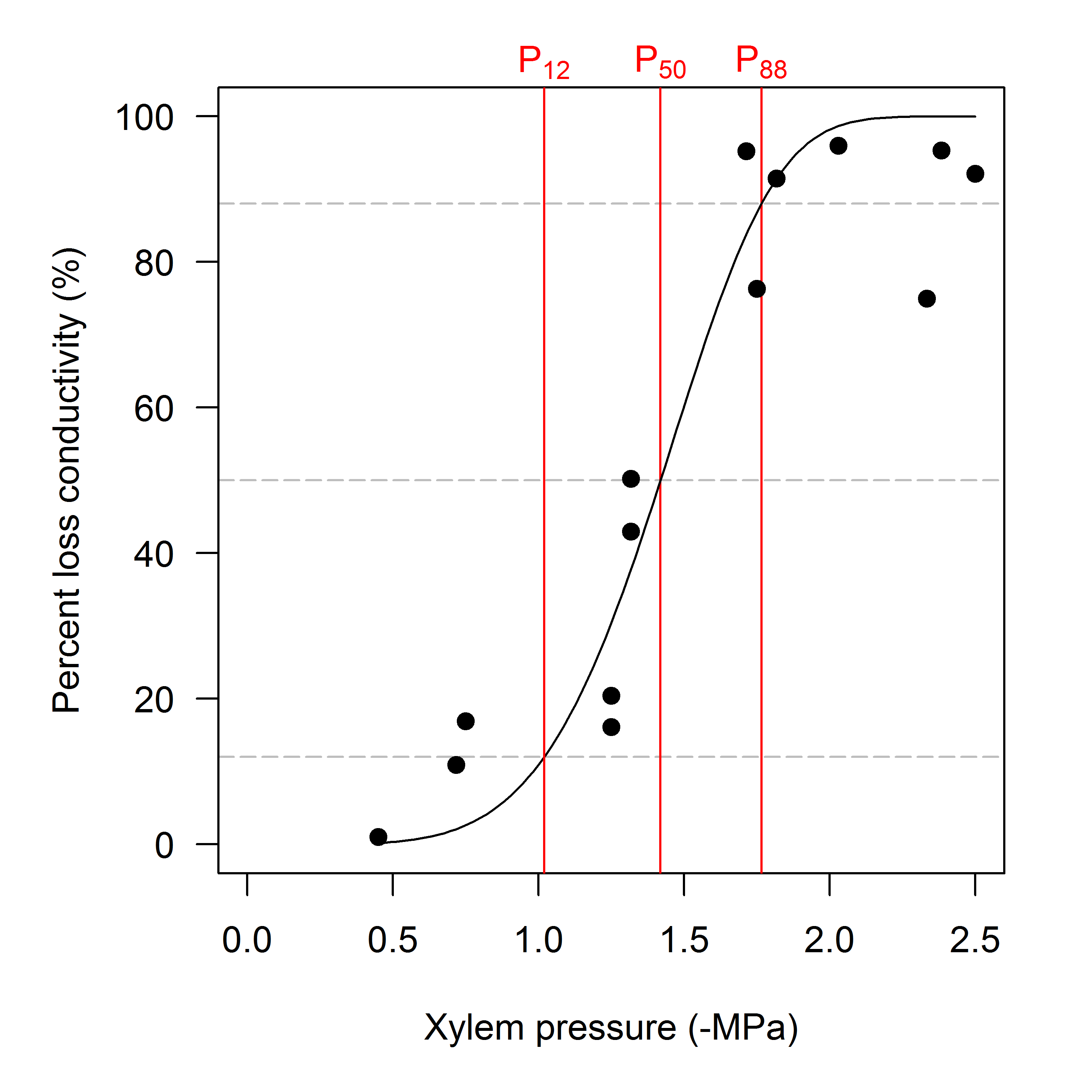Data are from a dehydration curve on Cochlospermum gillivraei.

In the following examples we focus on estimating P50 only, but note that any analysis can be quickly redone when some other parameter should be estimated. One of the strengths of the fitplc package is the use of the bootstrap to estimate confidence intervals of P50 as well as around the entire fitted curve. In the following example, we fit the Weibull curve to data from two species differing in sensitivity to stem xylem pressure, and make a simple plot. The calculation of the bootstrap confidence intervals is done by default, but can be switched off by the user if it is not needed to save time (though it only takes a few seconds to fit a curve and perform the bootstrap).

The example is shown in Fig. 2, where two species with very different vulnerability are compared in terms of their P50 and fitted curve. In this case, PLC at any xylem pressure is different between the species, as indicated by the non-overlapping 95% confidence intervals. Clearly, the P50's are also very different. Plots such as these are easy to construct with the default plotting routine, and refer to the online repository for the complete code to the examples.

Figure 2: Percent loss of conductivity (PLC) as a function of xylem pressure for two divergent species.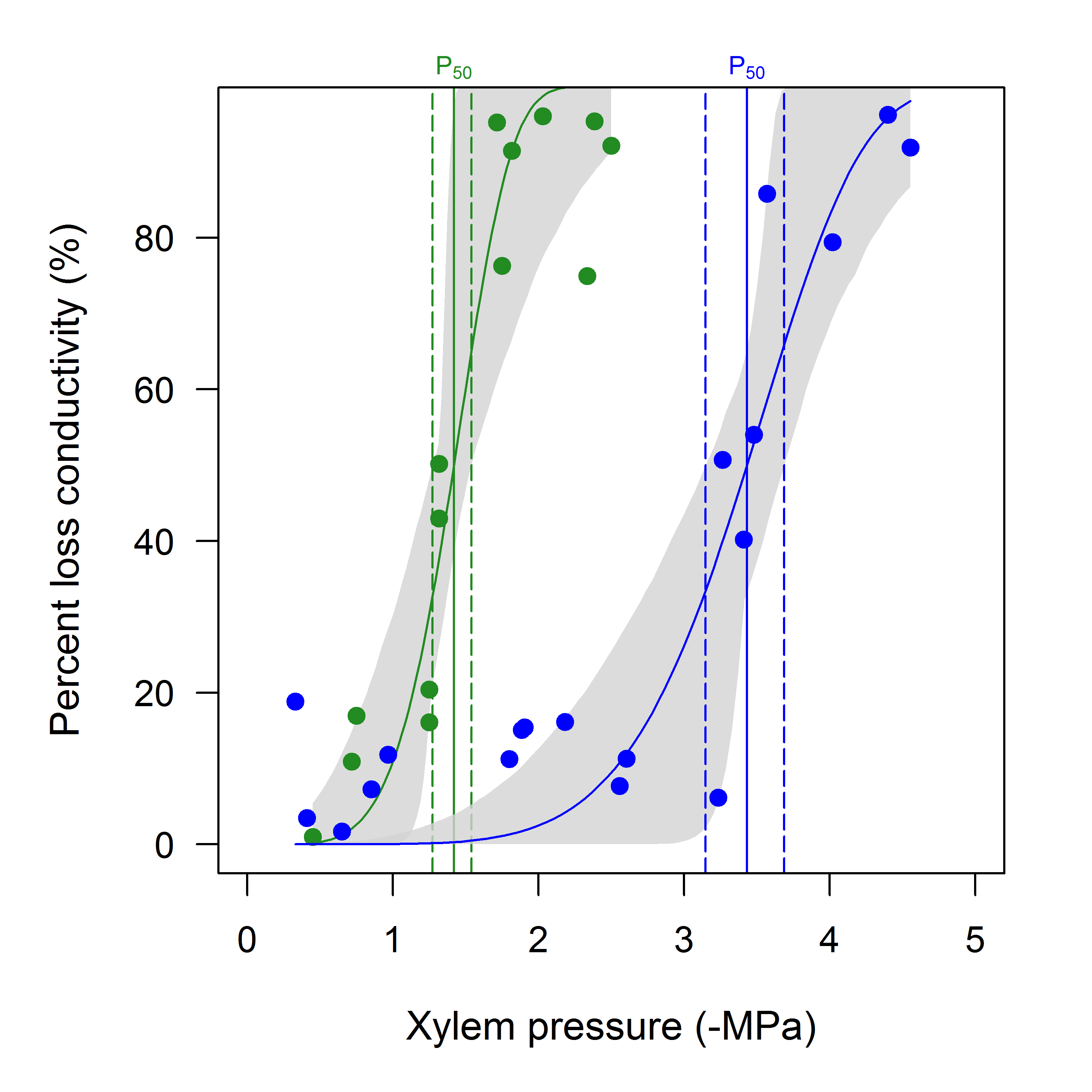Blue symbols and line : Prunus turneriana, green symbols and line : Cochlospermum gillivraei. Vertical dashed lines indicate the 95% confidence interval for P50 (estimated from the bootstrap in this example, though standard confidence intervals from non-linear regression can also be used). Note how the confidence intervals are not symmetric. The grey shaded area is the 95% bootstrapped confidence interval for the fitted curve.

When the conductivity has been scaled to a maximum value, we can use the fitplc function as shown in the previous examples. It is straightforward to scale conductivity measurements to the maximum observed for the dataset, however this approach is not robust to outliers. Another option is to scale the data relative to the average conductivity calculated for high water potentials (e.g. > -1 MPa). An example of the consequences of these two options is shown in Fig. 3.

Figure 3: Consequence of scaling assumption on curve fits.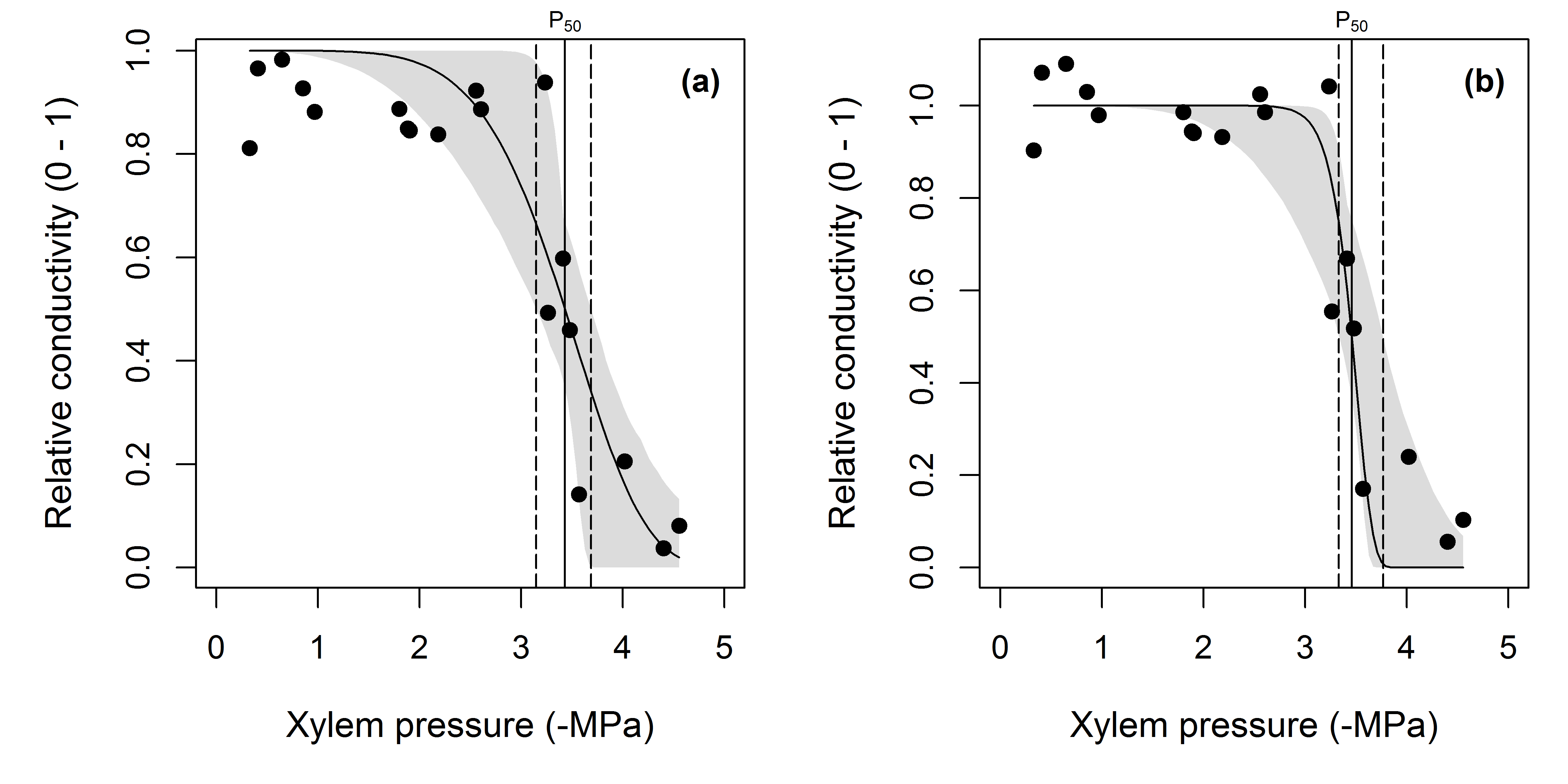(a) Standard fit to the Prunus data, note how the fitted curve overestimates relative conductivity at xylem pressures between 0 to -2 MPa. (b) The data were rescaled so that the average relative conductivity of the data where xylem pressure was between 0 and -1 MPa was 1 (instead of the maximum observed value being 1, as in panel a). Notice how the fit has improved as evidenced by the narrower confidence interval on the fitted curve as well as for P50. Estimates of P50 in panels (a) and (b) were not significantly different as evidenced by the overlapping confidence intervals (panel a, CI = 3.15 - 3.69, panel b CI = 3.33 - 3.77).

If the raw data have not been converted into PLC, but are available only as raw conductance (or conductivity) values, the fitcond function can be used. In this case, the user can either specify the maximum conductance for the dataset (perhaps measured independently or directly from the data, somehow), or a threshold water potential (in which case the maximum conductance will be calculated from the data where water potential is above this threshold). The user may also fit multiple curves at once (using fitconds); all other options to fitplc are supported in fitcond as well. In Fig. 4, we demonstrate the use of fitcond, and show a standard plot comparing three species.

The basic code to fit the example is shown below: here we fit the Weibull curve to conductivity directly, and scale the data by maximum conductivity calculated from the data where the water potential > -0.3 MPa (an arbitrary choice for this example).

fitc <- fitconds(mydata, group="Species", WP_Kmax = -0.3)

Figure 4: Example of curve fits to conductivity data.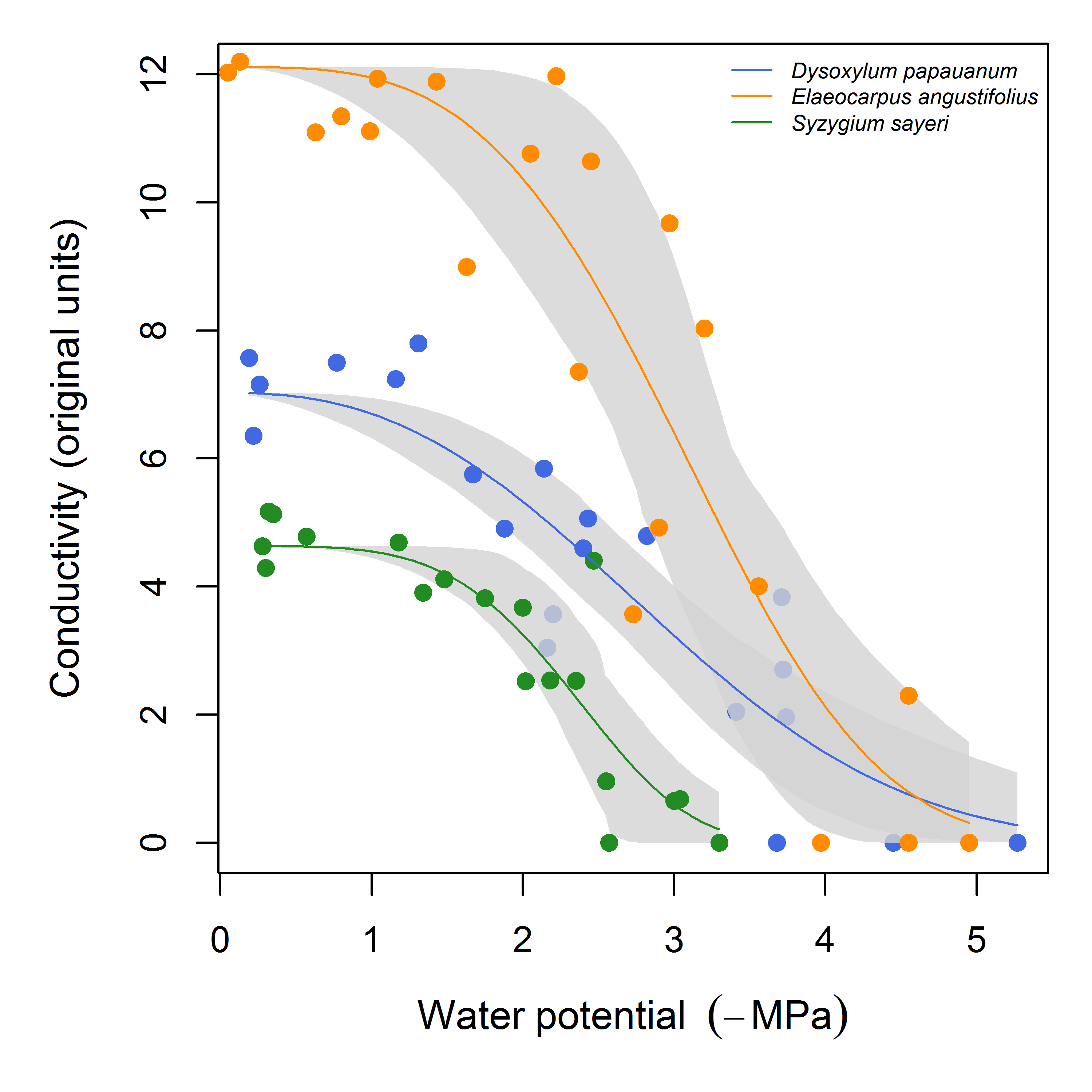In this example, the maximum conductivity was calculated from the data where water potential > -0.3 MPa. The grey areas are bootstrap confidence intervals for the fit. Estimates of P50 are omitted for clarity.

Finally we demonstrate the inclusion of a random effect. In both example datasets, multiple branches were measured for a species. The data points are not independent since the measurements on a single branch will be correlated. It is thus appropriate to fit a mixed-effects model. The fitted model is visualized in Fig. 5, including the mean response (i.e. the 'fixed effect') estimated by the model as well as the individual-level predictions for each branch (i.e. the random effects).

Fig. 5 shows the results from using fitplc when a random effect is included. Simplified code for these examples is shown below.

# Fit curves with 'individual' as a random effect (name of the variable in the dataframe)
fitran <- fitplc(mydata, random=individual)

# Standard plot, add predictions for random effect.
plot(fitran, plotrandom=TRUE)

Figure 5: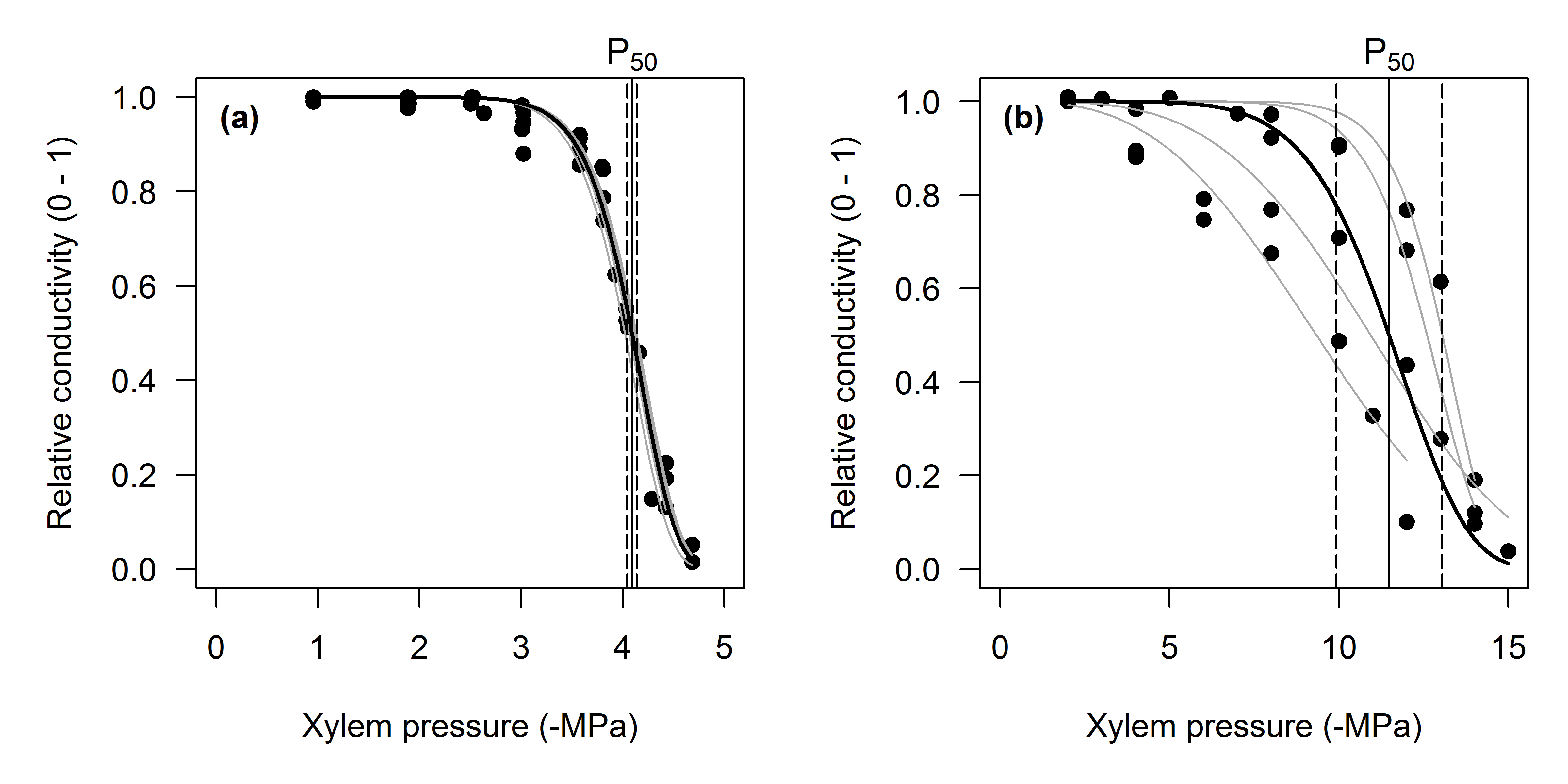(a) PLC curve for centrifuge-generated curves developed on Pinus radiata, fitted with a non-linear mixed effects model. Black solid line : the mean fit (i.e., the fixed effect), grey lines : predictions for the random effects. Also shown is the estimate of P50 with its 95% confidence interval. (b) Non-linear mixed effects model fit to data from Callitris glaucophylla. Lines as in panel a. Note the much larger variation between the curves, indicating a higher variance of the random effect for both P50 and the slope parameter.

Comparison of Weibull and sigmoidal models

We have so far demonstrated only the use of the Weibull model to estimate hydraulic parameters. The sigmoidal model is also implemented in the fitplc package, and can be fit with the following command:

fitsig <- fitplc(mydata, model = "sigmoidal")

A comprehensive comparison of the Weibull and sigmoidal models is well outside the scope of this paper. However, we found a few problems with the sigmoidal model, in particular the log-transformed version as presented by Pammenter and Van der Willigen (1998). The first problem, as reported by Ogle et al. (2009), is that the sigmoidal model does not guarantee that PLC is zero at P = 0; the PLC is often higher and depends on the overall response of PLC to P. In contrast, in the Weibull model, PLC = 0 at P = 0, which is more biologically reasonable. The second problem we found is that curves with a very steep response of PLC to P are poorly fit by the sigmoidal model (see Fig. 6a), but for other curves the fits are very comparable (Fig. 6b). Finally, the log-transformed version (Eq. 4) is very sensitive to very low values of conductivity, because values close to zero are large negative values when log-transformed, and may have a large impact on estimated coefficients (as we found with one example dataset; not shown). For these reasons we recommend the Weibull model as the default choice, but encourage more comprehensive comparisons.

Figure 6: Two comparisons of Weibull and sigmoidal fits.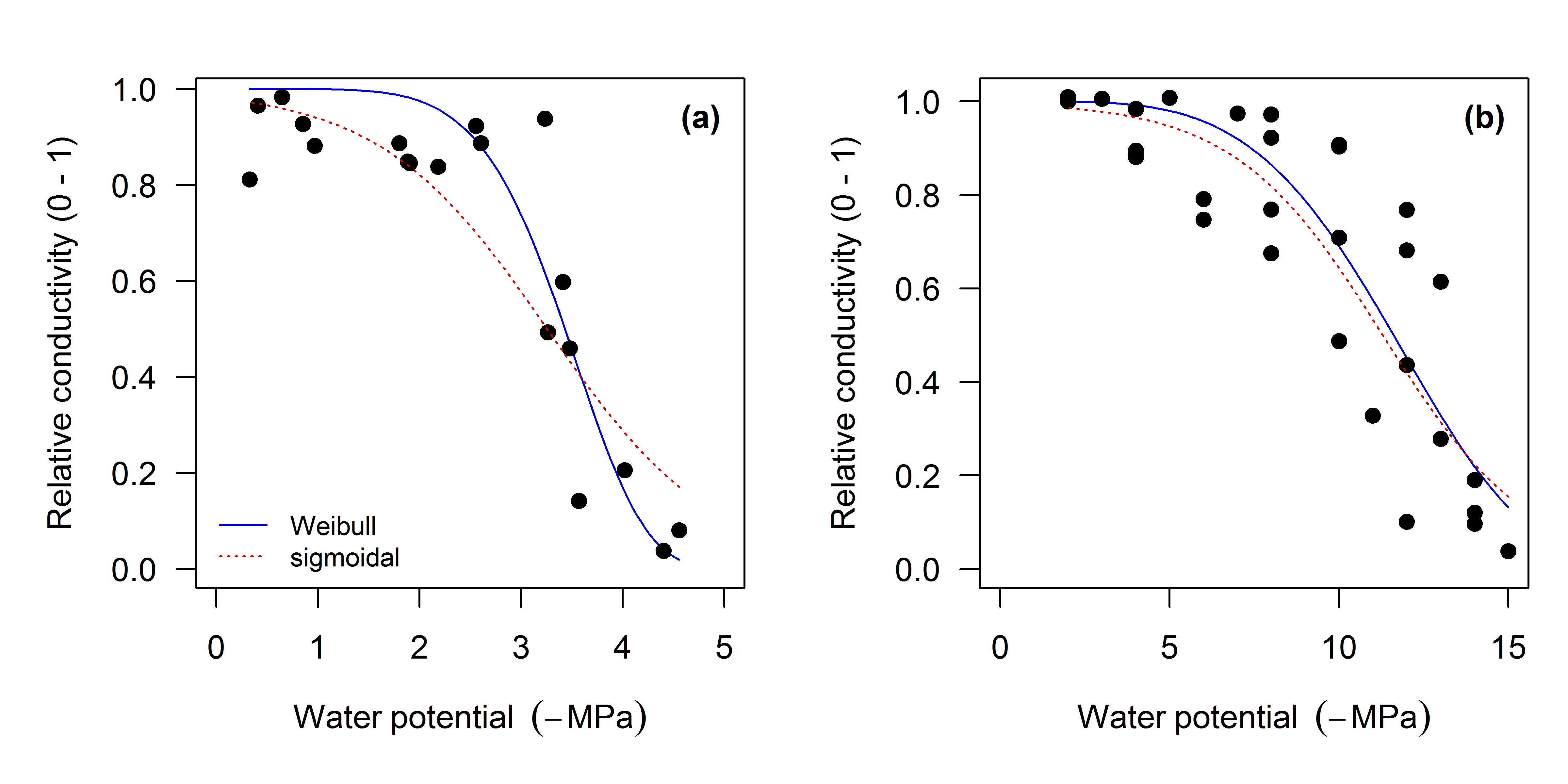In (a) (Prunus turneriana), the sigmoidal model provides a poor fit to PLC with a steep response to P. (b) For more gradual responses (i.e. lower Sx) the Weibull and sigmoidal models are very comparable.

Statistics

The fitplc package computes confidence intervals (CIs) of the fitted parameters with standard profiling methods or the bootstrap. It is well known that profiling standard errors in non-linear regression are often biased downward, thus underestimating the uncertainty of the fitted parameters. When possible, it is recommended to use the bootstrap resampling approach, as this provides reliable estimates of the CI. For the example datasets we have presented so far, we compared the width of the CI as reported by both methods (Table 1). As expected, the profiling method frequently underestimates the width of the CI, particularly for the slope parameter Sx. It is also worthwhile to point out that Sx often has very wide confidence intervals, demonstrating that this parameter is probably poorly constrained by the data, making meaningful comparisons between species difficult.

It is important to point out that in non-linear regression, the confidence interval cannot be simply computed from the standard error (as the usual approximation of 2 * SE). Instead, the confidence interval is computed based on profiling the likelihood functions of the parameters (Ritz and Streibig, 2008), and is frequently asymmetric (see Table 1). It is thus important to use confidence intervals for inferences, not the standard errors directly (e.g. as input to a t-test). For example, when 95% confidence intervals for P50 do not overlap between two species, one may conclude that P50 is significantly different between the two species (p < 0.05). For this reason, the fitplc package does not report the standard errors (although they can be extracted if really needed).

Table 1: Confidence intervals of the estimated parameters P50 and S50, using two independent methods : standard profiling (‘Norm’), and the bootstrap (‘Boot’).

Estimate

Norm - 2.5%

Norm - 97.5%

Boot - 2.5%

Boot - 97.5%

Eucalyptus tereticornis

S50

20.81

12.04

34.59

5.75

34.44

P50

4.31

3.82

4.8

3.74

6.33

Prunus turneriana

S50

61.93

32.9

NA

36.48

350.91

P50

3.43

3.21

3.64

3.15

3.69

Cochlospermum gillivraei

S50

124.92

67.86

NA

54.84

615.46

P50

1.42

1.31

1.52

1.27

1.54

Callitris glaucophylla

S50

12.5

7.91

19.23

7.73

21.05

P50

11.61

10.83

12.35

10.6

12.65

Dysoxylum papauanum

S50

30.63

20.71

43.84

20.56

45.32

P50

2.87

2.54

3.21

2.53

3.25

Elaeocarpus angustifolius

S50

39.32

24.85

66.71

26.02

92.96

P50

3.07

2.78

3.38

2.66

3.41

Syzygium sayeri

S50

64.26

36.35

132.53

47.44

165.69

P50

2.34

2.11

2.55

2.14

2.65

The confidence intervals are not usually symmetric around the mean, particularly for the bootstrap method. This indicates that the usual method of approximating the confidence interval as twice the standard error will often be incorrect. The width of the confidence interval as estimated by the bootstrap is generally larger than for the profiling method, but they generally agree quite well in magnitude. The upper bound for the confidence interval for Sx is occasionally unidentifiable (and indicated with 'NA'), and reaches very high values with either method in many cases.

## Discussion

We presented a new package that includes a range of statistical methods to fit hydraulic vulnerability curves, and report appropriate uncertainty measures. The main novelty of the approach is the use of the bootstrap to compute confidence intervals of the parameters, as well as around the entire fitted curve, and the use of (non-)linear mixed-effects models in cases where an ensemble of curves is measured. We discuss the difference in approach taken with the more common methods reported in the literature below, and provide some ideas for future work.

Uncertainty of the fitted vulnerability curve

Our implementation of a fitting routine for vulnerability curves is novel because it reports uncertainty of not just the fitted parameters, but also around the entire fitted curve, using the bootstrap. We do not claim that this is an entirely new method, indeed, this approach has been used frequently to report uncertainty of fitted non-linear curves. The bootstrap is widely used in ecology (Crowley, 1992), for example to report uncertainties in phylogenies (e.g., over 30 thousand citations to Felsenstein, 1985). We hope that the user-friendly implementation presented here will allow wider uptake in the field of plant hydraulics.

The use of the bootstrap to approximate the uncertainties differs from the fully Bayesian approach presented by Ogle et al. (2009). However, we are uncertain as to whether confidence intervals for the parameters, which form the basis of inference, will be necessarily different between the two approaches. It would be very interesting to compare statistical uncertainty from their approach with that of the bootstrap. The two approaches should not be seen as fully independent, as the non-parametric bootstrap can be viewed as an approximation to a Bayesian model (with a non-informative prior) (Rubin, 1981; Bååth, 2015). The advantage of the approach taken by Ogle et al. (2009) is that uncertainties at various hierarchical levels can be simultaneously estimated (for example, tree, split-plot, plot, and site).

Dealing with between-plant variability

The use of (non-)linear mixed-effects models (LME) is still quite rare in the analysis of response curves, although the technique is far from new (Peek et al., 2002). The most frequently used method to report hydraulic vulnerability parameters for a species when multiple individuals were measured (or for an individual with multiple branch measurements) is to fit curves to each individual, average the parameters and report the standard error for the mean (SE). In this way, species can be compared using the standard error and straightforward tests for significant differences (such as t-tests) (Pammenter and Van der Willigen, 1998). The LME approach has two distinct advantages. When curves are fit separately to each individual or branch, poor curve fits for some individuals (perhaps as the result of many missing values) will have a large effect on both the mean and the SE. With LME, these poor-fitting individuals are weighed less in the estimate of the mean response across all individuals (Pinheiro and Bates, 2006). In fact, it is even possible to include data from individuals where only one data point was measured. The second advantage is that predictions for individual level responses will have a higher accuracy with the LME approach compared to separate fitting (equivalent to shrinkage estimators discussed by Efron and Morris, 1977). Indeed, in the example with mixed-effects model that we showed (Fig. 5), the mixed-effects model fit resulted in more precise parameter estimates (lower standard errors; 0.78 vs. 0.89 for P50 and 5.59 vs. 7.11 for S in the example Callitris data). In addition, the goodness of fit was better, as judged by the RMSE (~ standard deviation of the residuals). The difference was small (< 4% in RMSE), but this difference may be larger when some individuals show a poor fit. We conclude that LME is a robust and practical method to allow for and estimate between-plant variability in hydraulic parameters.

Future work

We leave the door open for future improvements and contributions to the fitplc package. The code is hosted in an online repository, using git version control, allowing straightforward integration of contributions by others. One obvious improvement includes the possibility to include more models besides the Weibull and sigmoidal, to allow a test of the effect of model choice on parameter estimates and their uncertainties. Another possibility is the use of a non-parametric model that assumes no functional relationship (for example a GAM as used by Tobin et al. (2013)). It is interesting to note that estimates of P50 probably will depend on the model chosen to fit the data, but we are not aware of comprehensive comparisons made in the literature (though such comparisons are made for single datasets, see e.g. Guyot et al., 2012). Finally, we make no claim that the fitting method employed here is the best of all possible methods. We recognize the need to comprehensively compare different fitting methods, models to fit the data and methods to estimate uncertainty. Such a comparison is only possible when other methods are shared as open source implementations.

## Acknowledgements

We thank Markus Nolf, Danielle Creek, Chris Blackman and Rosana Lopéz for testing and suggesting features. This manuscript is entirely reproducible, including all figures and analyses. The code to generate this manuscript, which was written with the rmarkdown package, as well as all examples can be downloaded from : www.bitbucket.org/remkoduursma/fitplcpaper.

# Authors

#### Remko Duursma

Affiliation : Western Sydney University
Country : Australia
Biography : Senior Lecturer at the Hawkesbury Institute for the Environment

remkoduursma@gmail.com

#### Brendan Choat

Affiliation : Western Sydney University

# AttachmentsViews: 3685PDF: 185XML: 41